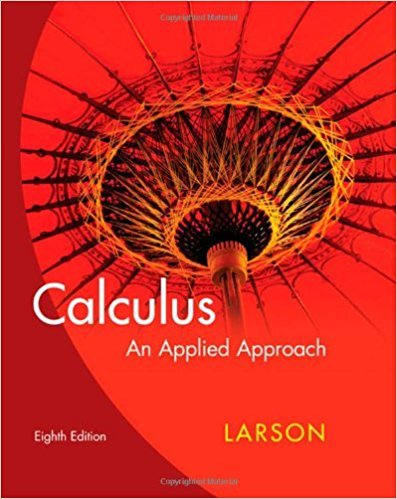×
×

# Solutions for Chapter 0.1: The Real Number Line and Order## Full solutions for Calculus: An Applied Approach | 8th Edition

ISBN: 9780618958252Solutions for Chapter 0.1: The Real Number Line and Order

Solutions for Chapter 0.1
4 5 0 376 Reviews
14
4
##### ISBN: 9780618958252

Since 38 problems in chapter 0.1: The Real Number Line and Order have been answered, more than 22533 students have viewed full step-by-step solutions from this chapter. This textbook survival guide was created for the textbook: Calculus: An Applied Approach , edition: 8. Calculus: An Applied Approach was written by and is associated to the ISBN: 9780618958252. Chapter 0.1: The Real Number Line and Order includes 38 full step-by-step solutions. This expansive textbook survival guide covers the following chapters and their solutions.

Key Calculus Terms and definitions covered in this textbook
• Branches

The two separate curves that make up a hyperbola

• Complex number

An expression a + bi, where a (the real part) and b (the imaginary part) are real numbers

• Difference of two vectors

<u1, u2> - <v1, v2> = <u1 - v1, u2 - v2> or <u1, u2, u3> - <v1, v2, v3> = <u1 - v1, u2 - v2, u3 - v3>

• Equivalent equations (inequalities)

Equations (inequalities) that have the same solutions.

• Function

A relation that associates each value in the domain with exactly one value in the range.

• Graph of an inequality in x and y

The set of all points in the coordinate plane corresponding to the solutions x, y of the inequality.

• Inverse reflection principle

If the graph of a relation is reflected across the line y = x , the graph of the inverse relation results.

• LRAM

A Riemann sum approximation of the area under a curve ƒ(x) from x = a to x = b using x1 as the left-hand endpoint of each subinterval

• Matrix element

Any of the real numbers in a matrix

• Observational study

A process for gathering data from a subset of a population through current or past observations. This differs from an experiment in that no treatment is imposed.

• Probability of an event in a finite sample space of equally likely outcomes

The number of outcomes in the event divided by the number of outcomes in the sample space.

• Product of complex numbers

(a + bi)(c + di) = (ac - bd) + (ad + bc)i

• Product rule of logarithms

ogb 1RS2 = logb R + logb S, R > 0, S > 0,

• Reduced row echelon form

A matrix in row echelon form with every column that has a leading 1 having 0’s in all other positions.

• Reflection through the origin

x, y and (-x,-y) are reflections of each other through the origin.

• Regression model

An equation found by regression and which can be used to predict unknown values.

• Right triangle

A triangle with a 90° angle.

• Right-hand limit of ƒ at x a

The limit of ƒ as x approaches a from the right.

• Venn diagram

A visualization of the relationships among events within a sample space.

• Vertical component

See Component form of a vector.

×